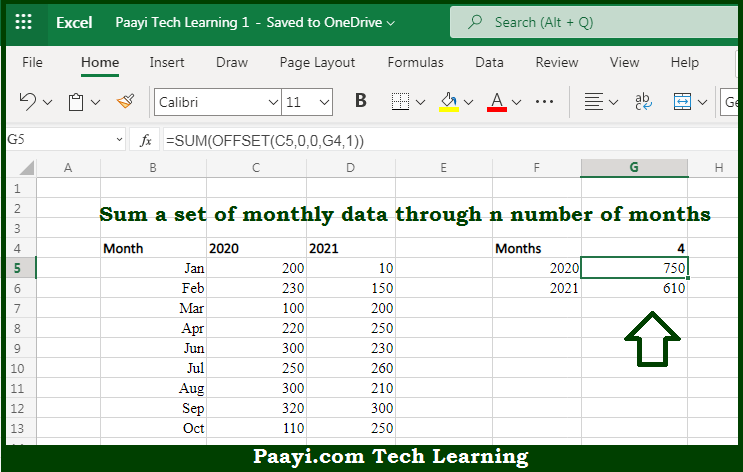# Learn How to SUM Through n Months in Microsoft Excel

Written by | 0 Comments | 397 Views

In this article, you will learn how to SUM various things in Microsoft Excel using a single/combination(s) of functions. You will also know how to SUM Through n Months and see the generic formula.

SUM Through n Months in Microsoft Excel

The main purpose of this formula is to sum a set of monthly data through n number of months. Here we will learn how to sum through n months in the workbook in Microsoft Excel. That implies, with the help of a formula based on the SQM and OFFSET function you can able sum a set of monthly data through n number of months. So, with the help of this formula, you can able to sum through n months in the workbook in Microsoft Excel.

General Formula to SUM Through n Months

=SUM(OFFSET(start,0,0,N,1))

The Explanation for the SUM Through n MonthsSo we know that with the help of the given formula above you can able to sum a set of monthly data through n number of months. Here we will learn how to sum through n months in the workbook in Microsoft Excel. As we know that we provided the monthly data in the example here for the given. The main purpose here is to sum values through a given number of months, provided in the G column. It is performed by feeding a reference constructed with the OFFSET function into the sum function. The OFFSET function lets you build a reference using a starting point, a row, and column offset, and a height and width. It should be noted that the OFFSET is handy in formulas that dynamically average or sum "last n values", "first n values", and so on. So, with the help of this formula, you can able to sum through n months in the workbook in Microsoft Excel.